Author: Oscar Cronquist Article last updated on May 04, 2022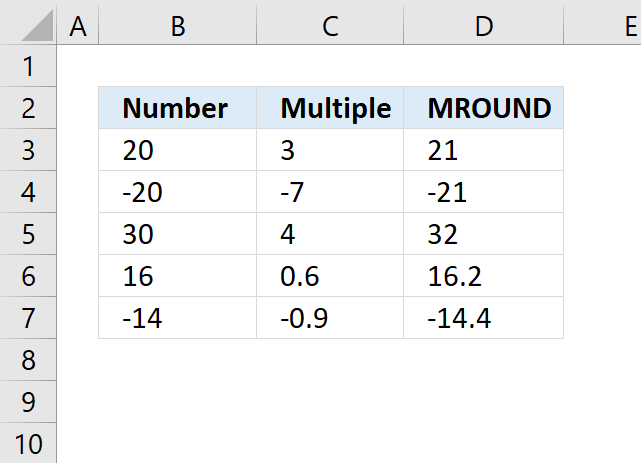The MROUND function calculates a number rounded to a given multiple.

Formula in cell D3:

=MROUND(B3,C3)

### Excel Function Syntax

MROUND(number, multiple)

### Arguments

 number Required. The value to round. multiple Required. The multiple you want to use.

Warning, the rounding direction is undefined for midpoint numbers.

## 1. How to round a date to the nearest given weekday?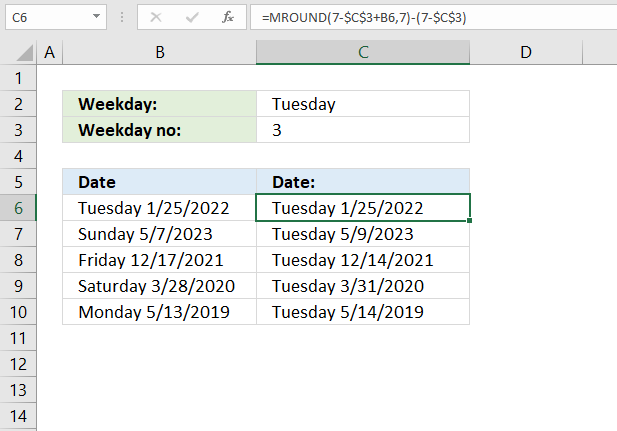The formula in cell C6 rounds the date in cell B6 to the nearest weekday based on the value in cell C2.

=MROUND(7-\$C\$3+B6,7)-(7-\$C\$3)

Cell C3 calculates the relative position of the weekday in cell C2 if the week begins on a Sunday.

Formula in cell C3:

MATCH(\$C\$2, {"Sunday"; "Monday"; "Tuesday"; "Wednesday"; "Thursday"; "Friday"; "Saturday"}, 0)

You can delete cell C3 and use this formula instead:

=MROUND(7-MATCH(\$C\$2, {"Sunday"; "Monday"; "Tuesday"; "Wednesday"; "Thursday"; "Friday"; "Saturday"}, 0)+B6, 7)-(7-MATCH(\$C\$2, {"Sunday"; "Monday"; "Tuesday"; "Wednesday"; "Thursday"; "Friday"; "Saturday"}, 0))

### Explaining formula in cell C3

#### Step 1 - Find the relative position of weekday

The MATCH function returns a number representing the relative position of a given value in an array or cell range.

MATCH(lookup_value, lookup_array, [match_type])

MATCH(\$C\$2,{"Sunday";"Monday";"Tuesday";"Wednesday";"Thursday";"Friday";"Saturday"},0)

becomes

MATCH("Tuesday", {"Sunday"; "Monday"; "Tuesday"; "Wednesday"; "Thursday"; "Friday"; "Saturday"}, 0)

and returns 3. Tuesday is the thrid value in the array.

#### Step 2 - Calculate Excel date

7-MATCH(\$C\$2,{"Sunday";"Monday";"Tuesday";"Wednesday";"Thursday";"Friday";"Saturday"},0)+B6

becomes

7-3+B6

becomes

7-3+44586 equals 44590

#### Step 3 - Round date

The MROUND function rounds a number to a given multiple.

MROUND(number, multiple)

MROUND(7-MATCH(\$C\$2,{"Sunday";"Monday";"Tuesday";"Wednesday";"Thursday";"Friday";"Saturday"},0)+B6,7)

becomes

MROUND(44590,7)

and returns 44590.

#### Step 4 - Calculate date of nearest weekday

MROUND(7-MATCH(\$C\$2,{"Sunday";"Monday";"Tuesday";"Wednesday";"Thursday";"Friday";"Saturday"},0)+B6,7)-(7-MATCH(\$C\$2,{"Sunday";"Monday";"Tuesday";"Wednesday";"Thursday";"Friday";"Saturday"},0))

becomes

44590 - (7-MATCH(\$C\$2,{"Sunday";"Monday";"Tuesday";"Wednesday";"Thursday";"Friday";"Saturday"},0))

becomes

44590-(7-3) equals 44586.

## 2. How to round a date to the nearest upcoming weekday?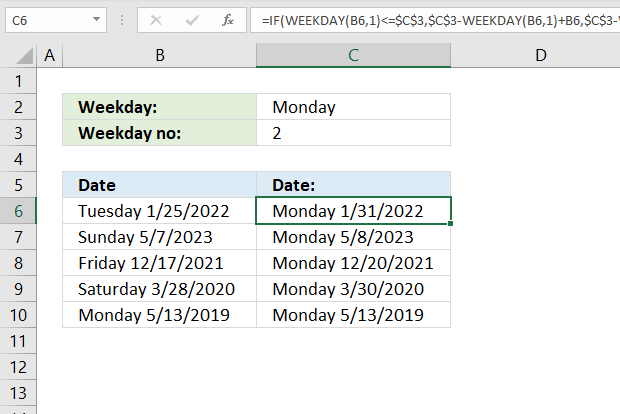Formula in cell C6:

=IF(WEEKDAY(B6, 1)<=\$C\$3, \$C\$3-WEEKDAY(B6, 1)+B6, \$C\$3-WEEKDAY(B6, 1)+B6+7)

### Explaining formula in cell C6

#### Step 1 - Check if weekday is less than or equal to value in C3

The WEEKDAY function returns a number representing the weekday.

WEEKDAY(serial_number,[return_type])

WEEKDAY(B6, 1)<=\$C\$3

becomes

WEEKDAY(44586, 1)<=\$C\$3

becomes

3<=\$C\$3

becomes

3<=2

and returns FALSE.

#### Step 2 - Evaluate IF function

The IF function returns one value if the logical test is TRUE and another value if the logical test is FALSE.

IF(logical_test, [value_if_true], [value_if_false])

IF(WEEKDAY(B6, 1)<=\$C\$3, \$C\$3-WEEKDAY(B6, 1)+B6, \$C\$3-WEEKDAY(B6, 1)+B6+7)

becomes

IF(FALSE, \$C\$3-WEEKDAY(B6, 1)+B6, \$C\$3-WEEKDAY(B6, 1)+B6+7)

and returns

\$C\$3-WEEKDAY(B6, 1)+B6+7

#### Step 3 - Calculate nearest upcoming weekday

\$C\$3-WEEKDAY(B6, 1)+B6+7

becomes

2-3+B6+7

becomes

2-3+44586+7

and returns 44592.

## 3. How to round time to the nearest hour?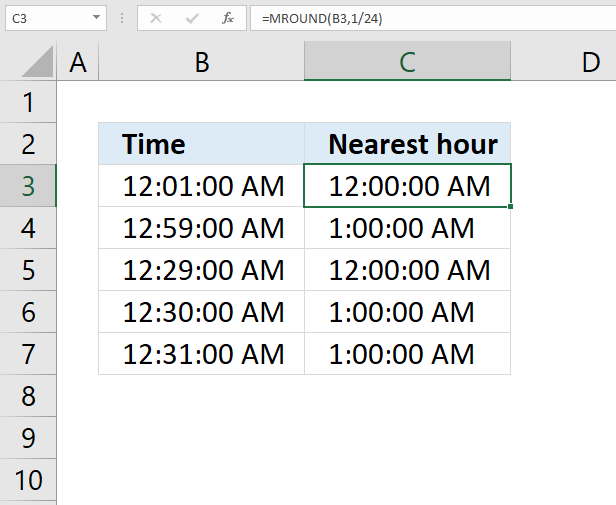Formula in cell C3:

=MROUND(B3,1/24)

### Explaining formula in cell C3

#### Step 1 - Excel time

Excel time is between 0 (zero) and 1. 24 hours is equal to 1.

1/24

An hour is equal to 1/24.

#### Step 2 - Round time to the nearest hour

MROUND(B3,1/24)

becomes

MROUND(0.000694444, 1/24)

becomes

MROUND(0.000694444, 0.041666667)

and returns 0 (zero) which is 12:00 AM.

## 4. How to round time to the nearest quarter of an hour?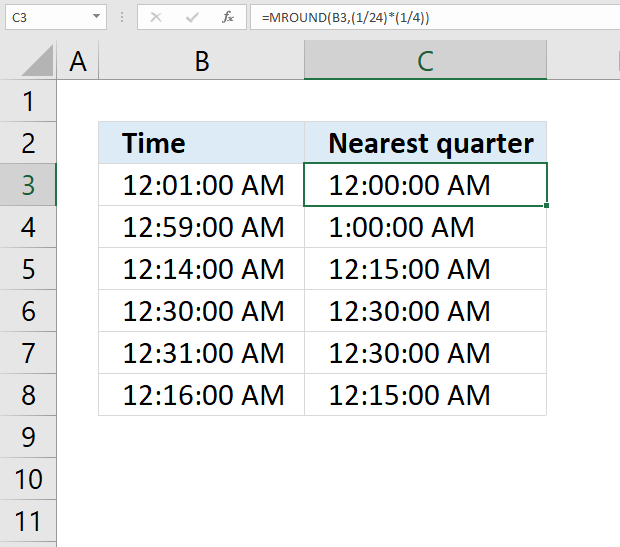Formula in cell C3:

=MROUND(B3,(1/24)*(1/4))

### Explaining formula in cell C3

#### Step 1 - Excel time

Excel time is between 0 (zero) and 1. 24 hours is equal to 1.

An hour is equal to 1/24. A quarter is 1/4 of an hour.

(1/24)*(1/4)

#### Step 2 - Round time to the nearest hour

MROUND(B3, (1/24)*(1/4))

becomes

MROUND(0.000694444, (1/24)*(1/4))

becomes

MROUND(0.000694444, 0.0104166666666667)

and returns 0 (zero) which is 12:00 AM.

### Get the Excel fileHow-to-use-the-MROUND-functionv2.xlsx